Using combination

Chapter 14 Class 11 Probability
Concept wise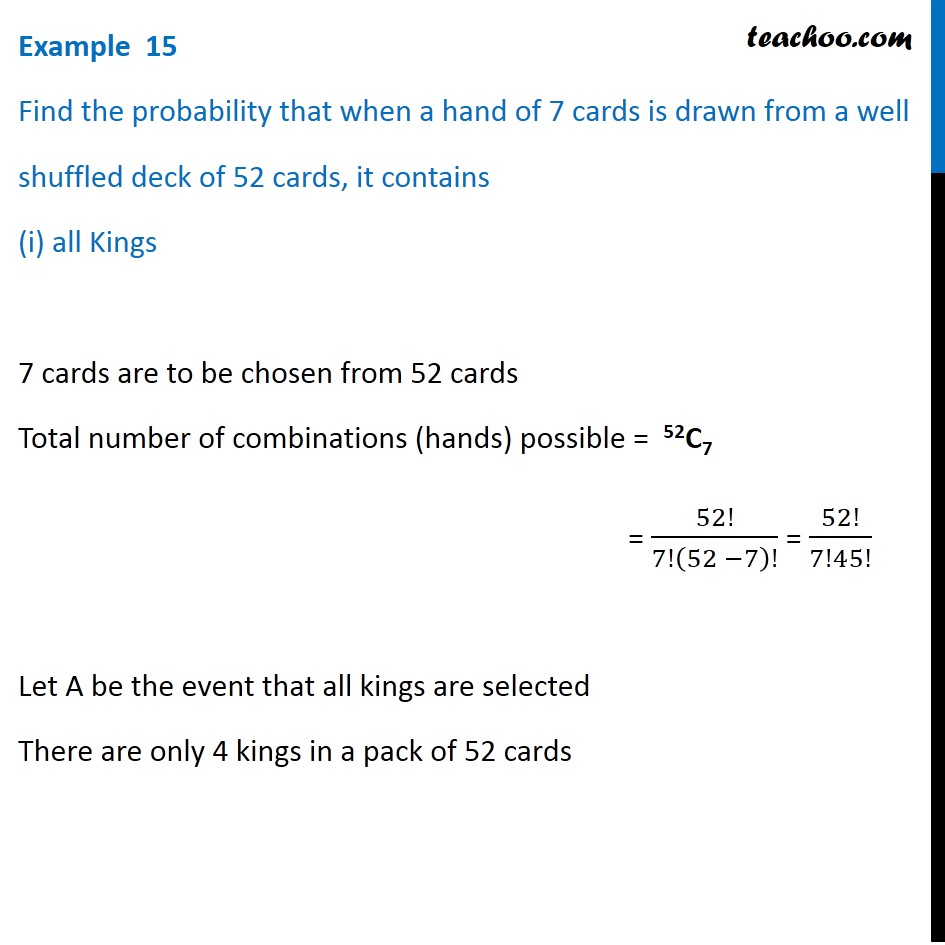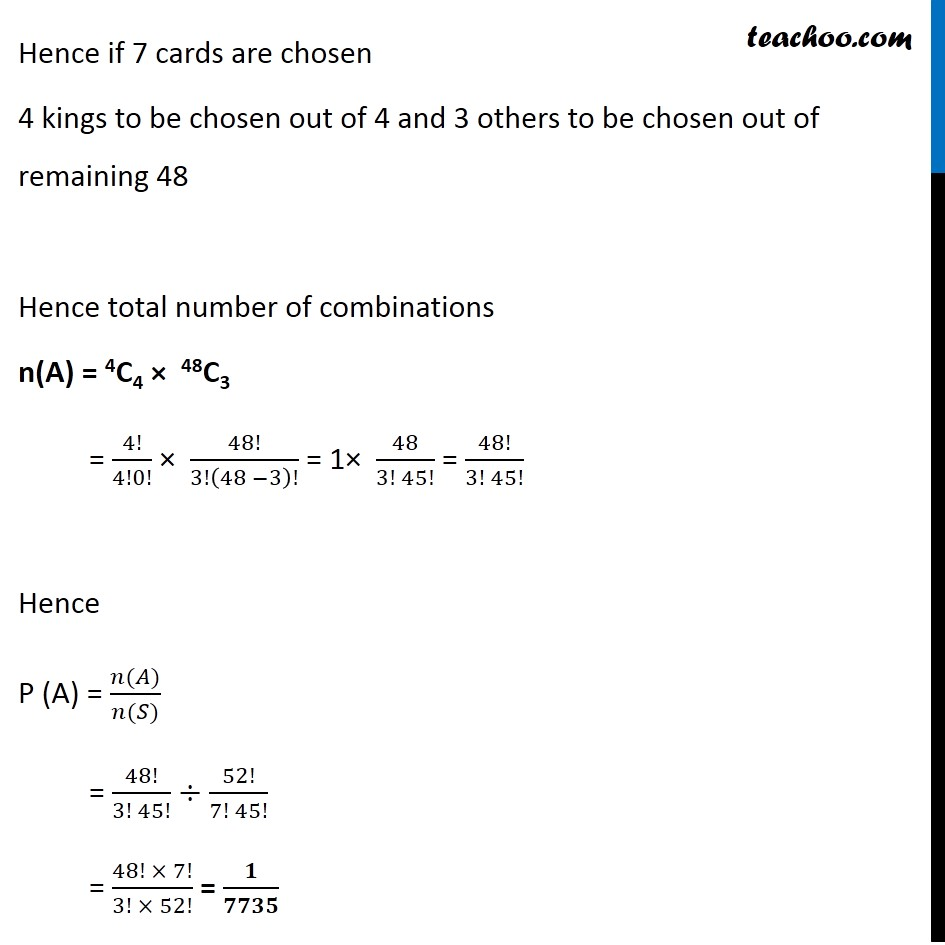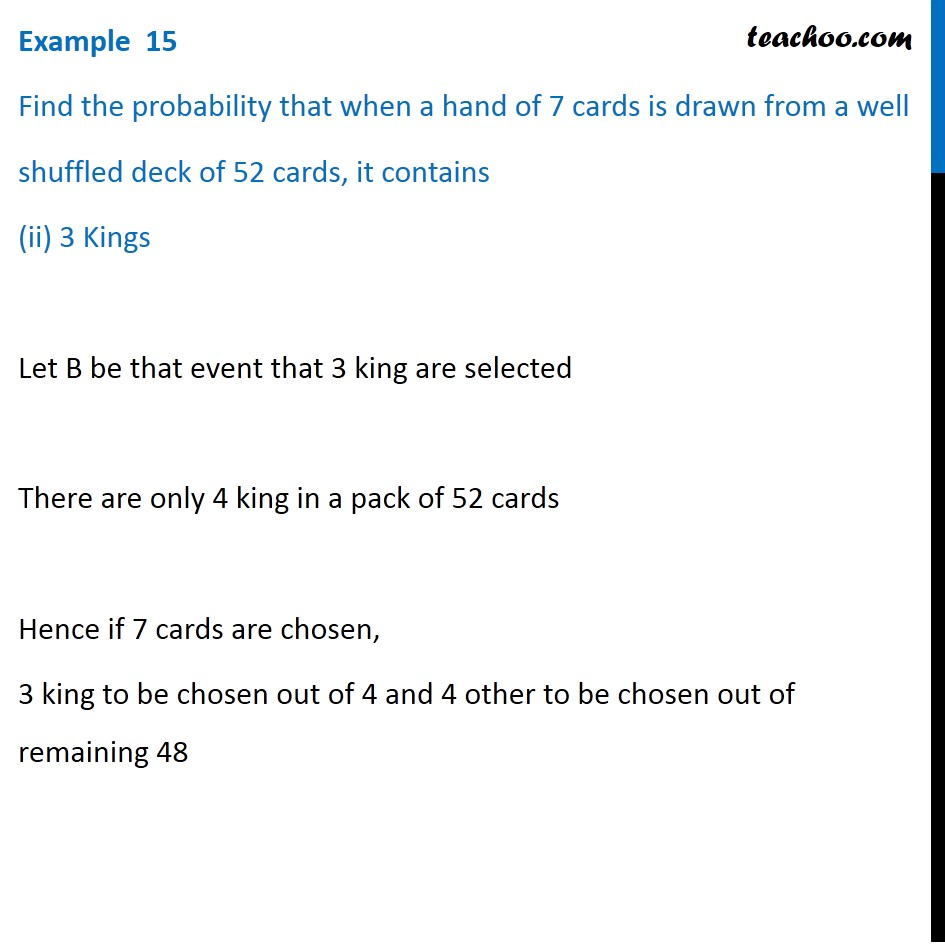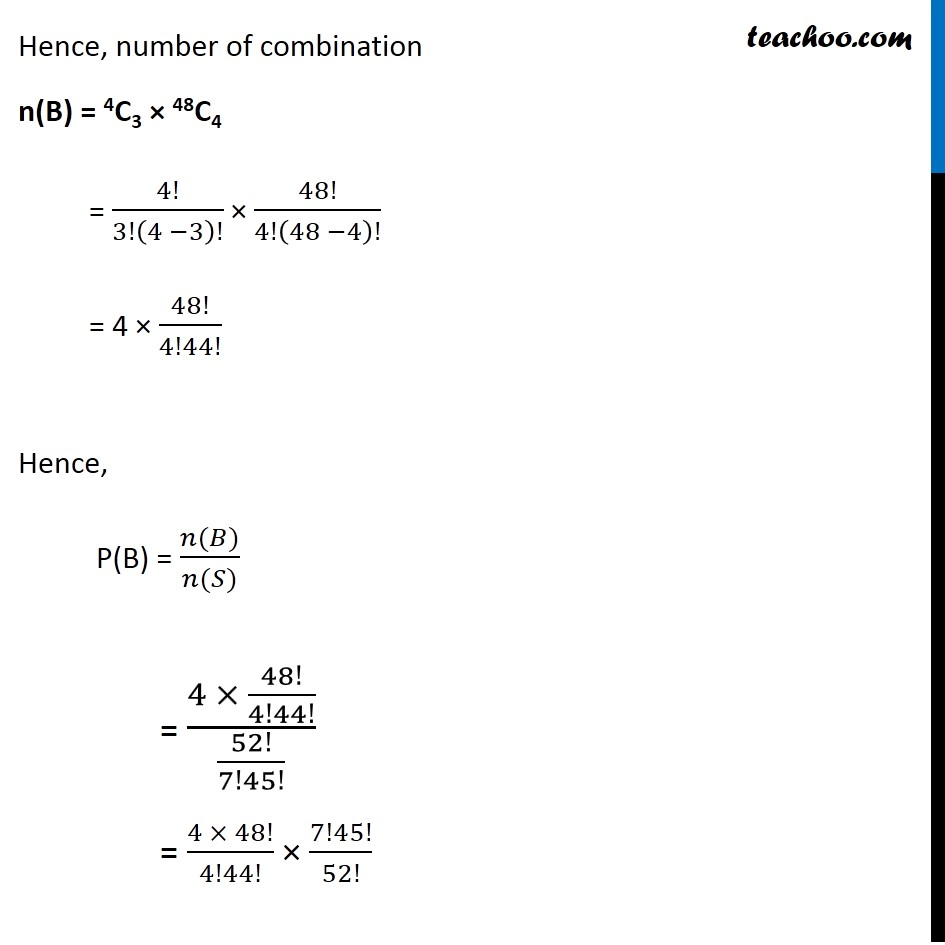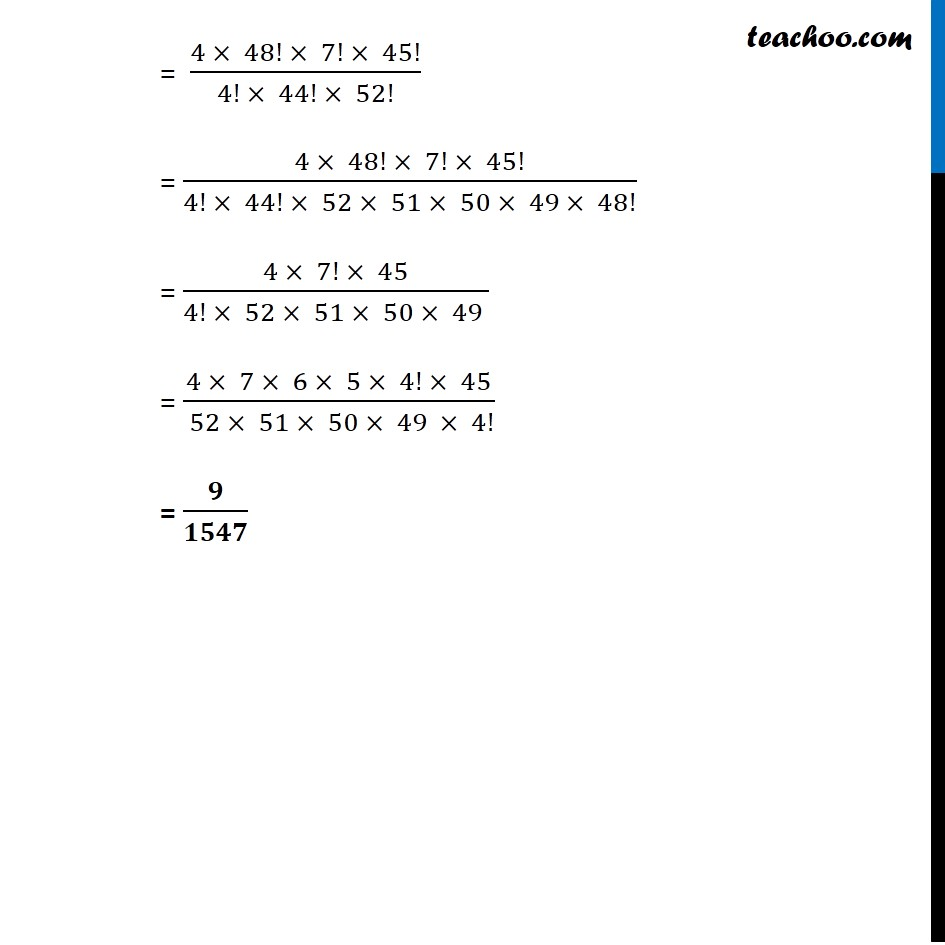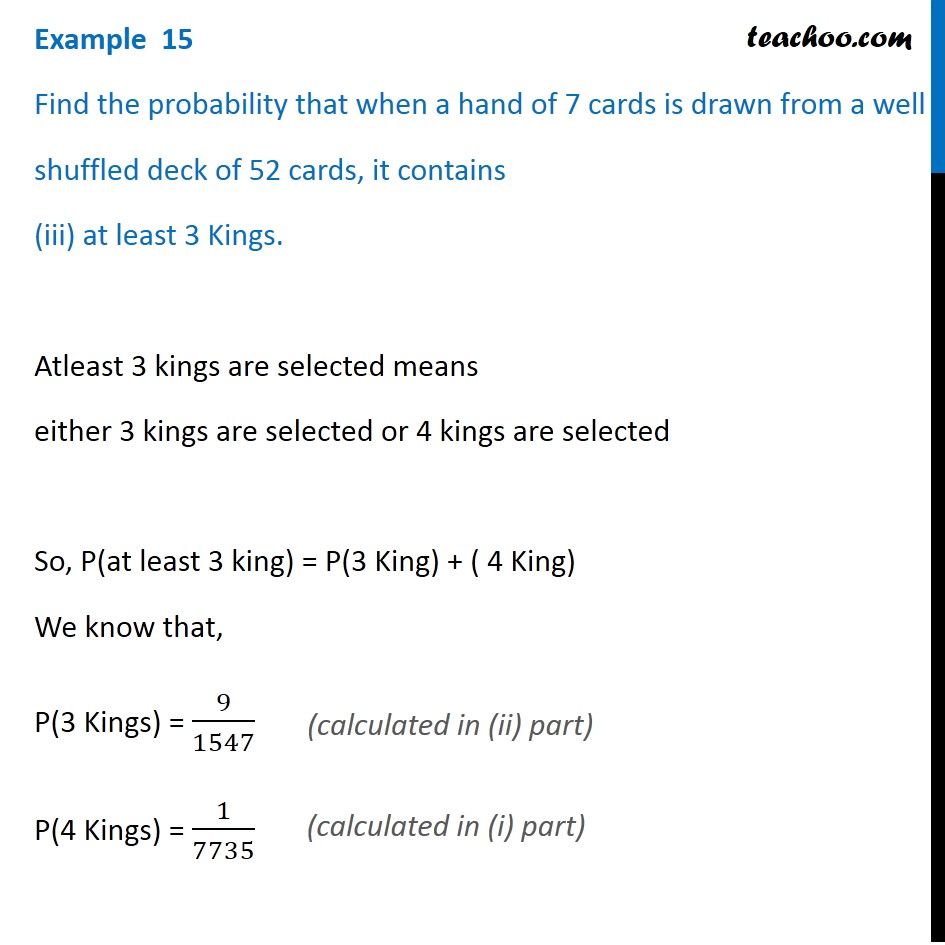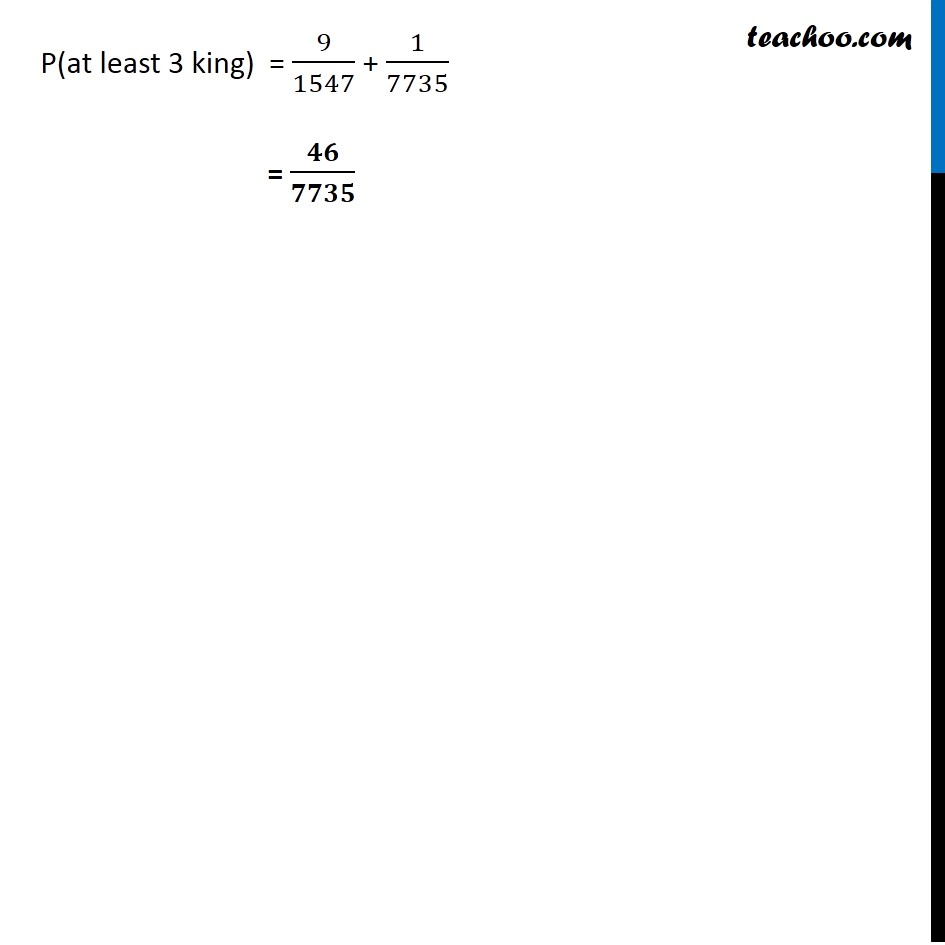Learn in your speed, with individual attention - Teachoo Maths 1-on-1 Class

### Transcript

Example 10 Find the probability that when a hand of 7 cards is drawn from a well shuffled deck of 52 cards, it contains (i) all Kings 7 cards are to be chosen from 52 cards Total number of combinations (hands) possible = 52C7 = 52!/7!(52 −7)! = 52!/7!45! Let A be the event that all kings are selected There are only 4 kings in a pack of 52 cards Hence if 7 cards are chosen 4 kings to be chosen out of 4 and 3 others to be chosen out of remaining 48 Hence total number of combinations n(A) = 4C4 × 48C3 = 4!/4!0! × 48!/3!(48 −3)! = 1× 48/(3! 45!) = 48!/(3! 45!) Hence P (A) = (𝑛(𝐴))/(𝑛(𝑆)) = 48!/(3! 45!) ÷ 52!/(7! 45!) = (48! × 7!)/(3! × 52!) = 𝟏/𝟕𝟕𝟑𝟓 Example 10 Find the probability that when a hand of 7 cards is drawn from a well shuffled deck of 52 cards, it contains (ii) 3 Kings Let B be that event that 3 king are selected There are only 4 king in a pack of 52 cards Hence if 7 cards are chosen, 3 king to be chosen out of 4 and 4 other to be chosen out of remaining 48 Hence, number of combination n(B) = 4C3 × 48C4 = 4!/3!(4 −3)! × 48!/4!(48 −4)! = 4 × 48!/4!44! Hence, P(B) = (𝑛(𝐵))/(𝑛(𝑆)) = (4 × 48!/4!44!)/(52!/7!45!) = (4 × 48!)/4!44! × 7!45!/52! = (4 × 48! × 7! × 45!)/(4! × 44! × 52!) = (4 × 48! × 7! × 45!)/(4! × 44! × 52 × 51 × 50 × 49 × 48!) = (4 × 7! × 45)/(4! × 52 × 51 × 50 × 49 ) = (4 × 7 × 6 × 5 × 4! × 45)/( 52 × 51 × 50 × 49 × 4!) = 𝟗/𝟏𝟓𝟒𝟕 Example 10 Find the probability that when a hand of 7 cards is drawn from a well shuffled deck of 52 cards, it contains (iii) at least 3 Kings. Atleast 3 kings are selected means either 3 kings are selected or 4 kings are selected So, P(at least 3 king) = P(3 King) + ( 4 King) We know that, P(3 Kings) = 9/1547 P(4 Kings) = 1/7735 (calculated in (ii) part) (calculated in (i) part) P(at least 3 king) = 9/1547 + 1/7735 = 𝟒𝟔/𝟕𝟕𝟑𝟓